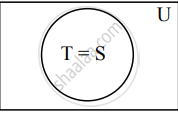# Represent the truth of the following statement by the Venn diagram. All teachers are scholars and scholars are teachers. - Mathematics and Statistics

Sum

Represent the truth of the following statement by the Venn diagram.

All teachers are scholars and scholars are teachers.

#### Solution

Let U : The set of all human beings.
T : The set of all teachers.
S : The set of all scholarsThe above Venn diagram represents truth of the given statement, T = S

Concept: Venn Diagrams
Is there an error in this question or solution?
Chapter 1: Mathematical Logic - Exercise 1.10 [Page 27]

Share